﻿ 基于滚动时域的无人机动态航迹规划
«上一篇文章快速检索 高级检索

 智能系统学报2018, Vol. 13Issue (4): 524-533  DOI: 10.11992/tis.2017080310

### 引用本文WANG Wenbin, QIN Xiaolin, ZHANG Lige, et al. Dynamic UAV trajectory planning based on receding horizon[J]. CAAI Transactions on Intelligent Systems, 2018, 13(4): 524-533. DOI: 10.11992/tis.201708031.### 文章历史

1. 中国科学院 成都计算机应用研究所，四川 成都 610041;
2. 中国科学院大学 计算机与控制学院，北京 100080;
3. 广州大学 智能软件研究院，广东 广州 510006

Dynamic UAV trajectory planning based on receding horizon
WANG Wenbin1,2, QIN Xiaolin1,2,3, ZHANG Lige1,2, ZHANG Guohua1,2
1. Chengdu Institute of Computer Applications, Chinese Academy of Sciences, Chengdu 610041, China;
2. School of Computer and Control Engineering, University of Chinese Academy of Sciences, Beijing 100080, China;
3. Academy of Intelligent Software, Guangzhou University, Guangzhou 510006, China
Abstract: Using receding horizon control and fast particle swarm optimization (RHC-FPSO), in this paper, we propose an algorithm for unmanned aerial vehicle (UAV) trajectory planning with dynamic constraints. We introduce the cost map method based on the VORONOI graph to estimate the distance from the end point of the trajectory to the target point. Using the concept of receding horizon control and the artificial potential field method, the path planning problem is transformed into an optimization problem, with the minimum distance and other performance indicators as cost functions. We design the evaluation function criteria based on the evaluation criteria and obtain the solution using a particle swarm optimization algorithm with variable weight. To address the problem in which a UAV approaches a danger zone, we introduce a repulsion field into the cost function to ensure safety. The simulation results show that the proposed method can effectively avoid obstacles within the constraint conditions and perform dynamic calculations in a complicated environment.
Key words: trajectory planning    receding horizon control    VORONOI graph    variable weight    particle swarm optimization    artificial potential field

1 相关工作 1.1 滚动时域控制原理

RHC的基本思想：假设当前时间点为 $k$ ，在有限时域 $[k,k + {H_p}]$ ，通过使某一性能指标最优化来确定其未来的控制作用，选择第一个最优控制输入作为当前的控制输入，在下一时刻 $k + 1$ ，计算 $[k + 1,k + {H_p} + 1]$ 的控制输入，重复以上过程，直到任务完成。具体步骤如下：

1) 根据当前状态 ${{x}}(k)$ ，考虑当前约束和未来约束，计算未来时域 ${H_p}$ 内的输入 $[{{u}}(k)\,\,{{u}}(k+1)\,\, \cdots$ ${{u}}(k + {H_p})]$

2) 选择控制时域 ${H_e}$ ，即 $[{{u}}(k)\,\,{{u}}(k+1)\,\, \cdots \,\,{{u}}(k + {H_e})]$ 作为当前的输入。

3) 当到达 $k + {H_e} + 1$ 时刻时，测量当前状态 $x(k + {H_e} + 1)$

4) 当 $k + {H_e} + 1$ 时刻，在有限时域 $[{{u}}(k + {H_e} + 1),$ ${{u}}(k + {H_e} + {H_p} + 1)]$ 内重复步骤1)～3)直到达到目标。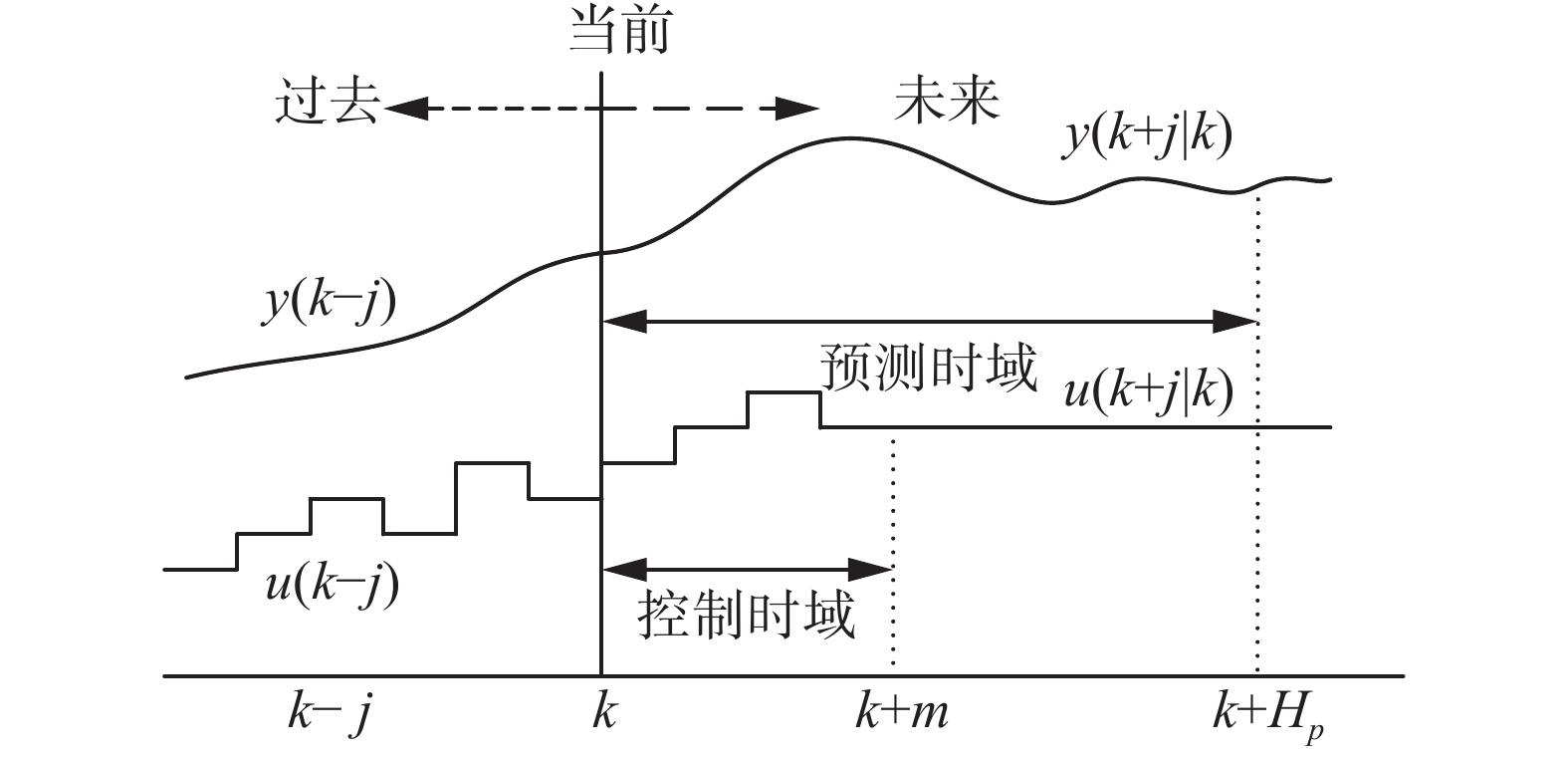Download: 图 1 滚动时域控制示意图 Fig. 1 The diagram of receding horizon control
1.2 人工势场法

 $U(x) = {U_{\rm{att}}}(x) + {U_{\rm{rep}}}(x)$ (1)

 ${U_{\rm{att}}}(x) = \frac{1}{2}{k_{\rm{att}}}{d^2}(x,{x_g})$ (2)

 ${U_{\rm{rep}}}(x) = \left\{ {\begin{array}{l} {\displaystyle\frac{1}{2}{k_{\rm{rep}}}(\frac{1}{{d(x,{x_0})}} - \frac{1}{{{d_n}}})}, \quad {d(x,{x_o}) \leqslant {d_n}}\\ 0 , \quad {d(x,{x_o}) > {d_n}} \end{array}} \right.$ (3)

2 问题描述

 ${{x}}(k + 1) = {{Ax}}(k) + {{Bu}}(k)$ (4)
 ${{y}}(k) = {{Cx}}(k) + {{Du}}(k) \in {{\gamma }}(k)$ (5)

 $J = \sum\limits_{k = 0}^\infty {l({{u}}(k),{{x}}(k),{\chi _F})}$ (6)

 $\begin{array}{c}{J^*} = \mathop {\min }\limits_{u(.)} \\sum\limits_{j = 0}^{{H_p}} {l({{u}}(k + j|k),{{x}}(k + j|k),{\chi _F})} + \\f({{x}}(k + {H_p} + 1),{\chi _F})\} \end{array$ (7)
 $\begin{array}{c}{{x}}(k + j + 1|k) = {{Ax}}(k + j|k) + {{Bu}}(k + j|k)\\j = 0, 1,\cdots ,{H_p}\end{array}$ (8)
 $\begin{array}{c}{{y}}(k + j|k) = {{Cx}}(k + j|k) + {{Du}}(k + j|k) \in {{\gamma }}(k)\\j = 0,1, \cdots ,{H_p}\end{array}$ (9)

3 RHC-FPSO航迹规划

3.1 代价图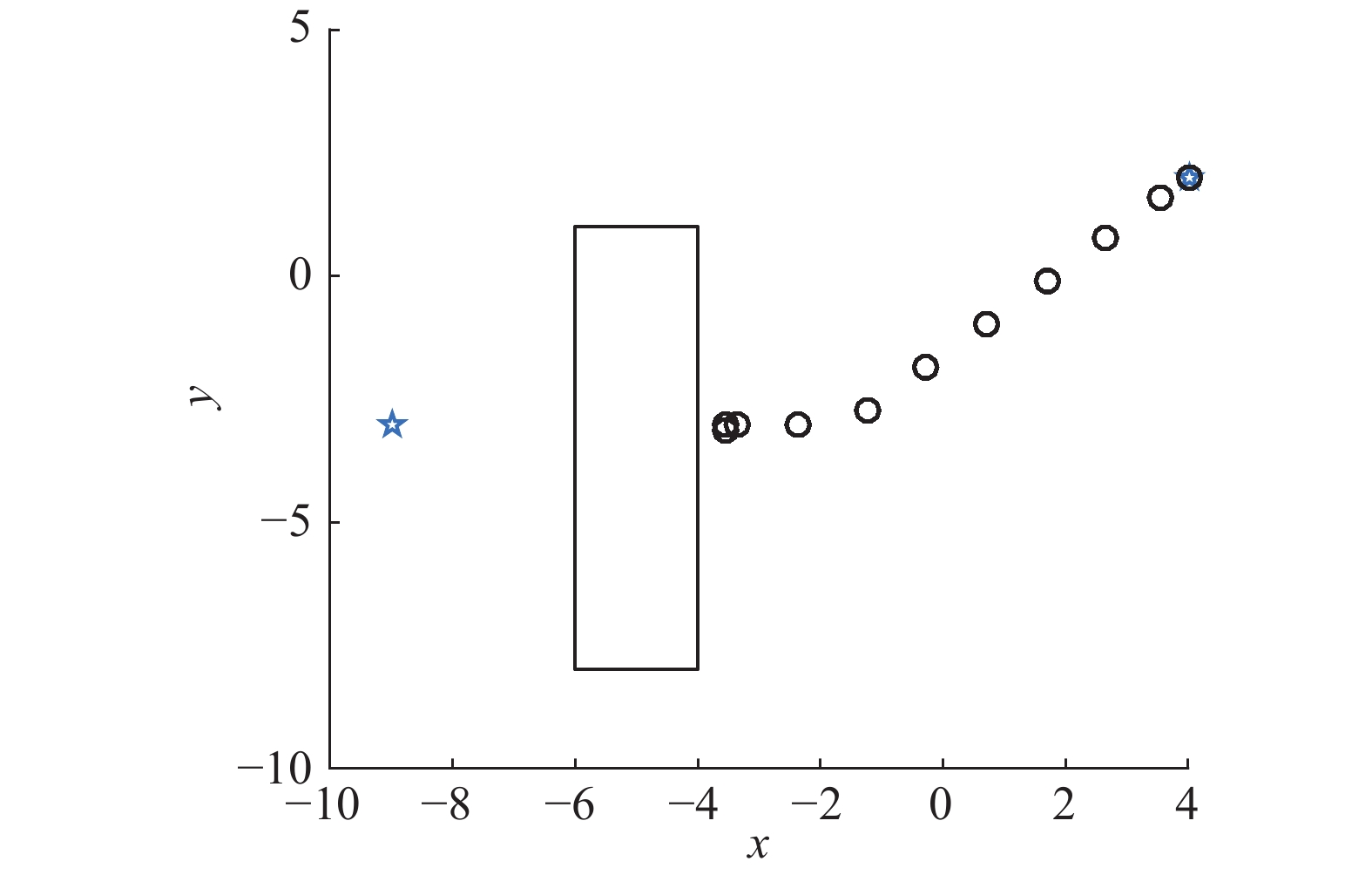Download: 图 2 不含代价图的航迹规划示意图 Fig. 2 The diagram of trajectory planning without cost map

 $f({{x}}(k + {H_p} + 1),{\chi _F})) = d({{x}}(k + {H_p} + 1),{{{x}}_{\rm{vis}}}) + {C_{\rm{vis}}}$ (10)

3.2 基于RHC-FPSO在线航迹规划

1) 对于可行输入来说，根据式(3)，代价小的输入优于代价大的输入；

2) 对于不可行航迹来说，约束违背小的输入优于约束违背大的输入；

3) 对于可行输入与不可行输入，可行输入总是优于不可行输入；

 $F({{u}}) = \left\{ {\begin{array}{l}{C({{u}}), \quad {\text{可行解}}}\\{\rm{BigM }}+ C, \quad {\text{不可行解}}\end{array}} \right.$ (11)

 $C = \sum\limits_{i = 1}^{{H_p}} {({w_1}f({l_i}) + {w_2}g({v_i}) + {w_3}h({a_i}))}$ (12)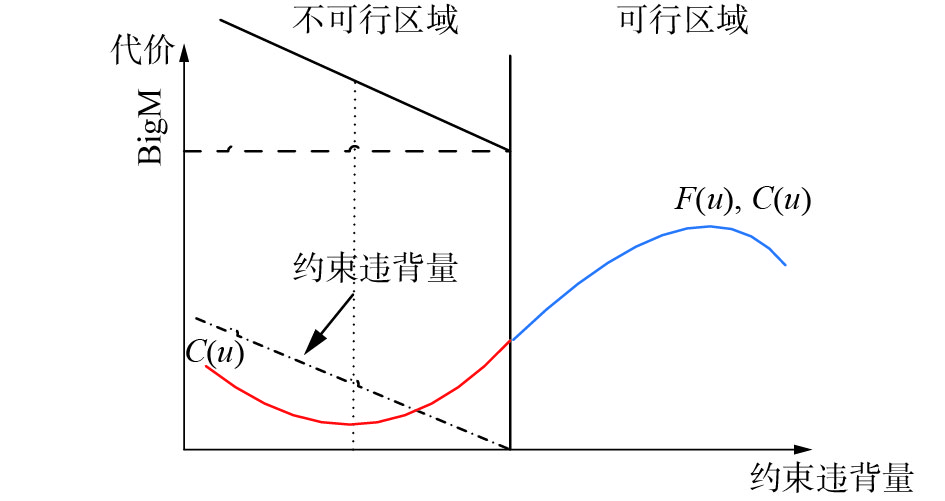Download: 图 3 评价函数 $F(u)$ 图像 Fig. 3 The image of evaluation function F(u)

 $\left\{ {\begin{array}{l}\begin{array}{c}\!\!\!\!\!\!\!\!{{{v}}_i}(k + 1) = {{w}}(k){{{v}}_i}(k) + {c_1}{r_1}({{{P}}_i}(k) - {{{x}}_i}(k)) +\\\!\!\!\!\!\!\! \!\!\!\!\!{c_2}{r_2}({{G}}(k) - {{{x}}_i}(k))\end{array}\\\!\!\!\!{{{{x}}_i}(k + 1) = {{{x}}_i}(k) + w{{{v}}_i}(k)}\end{array}} \right.$ (13)

 $w(k) = {w_{\max }} - \frac{{{w_{\max }} - {w_{\min }}}}{{{{\rm{iter}}_{\max }}}}k$ (14)

 ${w_{\max }}(k,i) = 1 - \frac{{{F_{k,i}}(u)}}{{\mathop {\max }\limits_i ({F_{k,i}}(u))}}$ (15)

3.3 基于RHC-APF在线航迹规划

3.1节介绍的方法中，引入的VORONOI图，构建比较复杂，而且当环境改变的时候，需要重新计算，不利于实时航迹规划。本节结合APF实时计算和计算简单的特点，提出RHC-APF算法。

1) 基于4.1提出的代价图，会导致无人机靠近障碍物飞行。按照APF的思想，让障碍物给无人机一个斥力场，因此此时的目标函数可以表示为式(16)的形式：

 $J = {J^*} + {U_{\rm{rep}}}$ (16)

2) 代价图的计算会增加计算负担，而且不利于在线规划，因为当环境发生改变时，需要重新计算代价图，特别突发事件，会降低实时性。人工势场法只需增加障碍物的一个势场，当目标发生改变时，也只需改变目标函数的势场，并且势场的计算非常简单。将RHC和APF结合后能够克服APF的不足，同时增加了实时性。此时的目标函数为

 $J = {U_{\rm{att}}} + {U_{\rm{rep}}}$ (17)
3.4 算法实现流程

1) 首先初始化无人机参数，设置最大速度、最大加速等限制，设置滚动时域，以及无人机采样间隔。

2) 初始化粒子群P，包括粒子的位置 ${{{x}}_i} =$ $({x_{i,1}},{x_{i,2}}, \cdots ,{x_{i,{H_p}}})$ 和速度 ${{{v}}_i} = ({v_{i,1}},{v_{i,2}}, \cdots ,{v_{i,{H_p}}})$ ，按照表1设置相关参数。表 1 仿真参数和值 Tab.1 Simulation parameters and values

3) 按照式(11)和式(12)分别计算每个粒子的适应度和约束违背量。

4) 更新粒子群最优解和每个粒子的最优解。

5) 如果粒子满足约束条件，按照式(13)和式(14)更新粒子的位置和速度。否则，按照式(13)和式(15)更新粒子的位置和速度。

6) 如果粒子群不满足终止条件，转3)。

7) 取最优结果 ${{{x}}_{\rm{best}}} = ({x_{\rm{best},1}},{x_{\rm{best},2}}, \cdots ,{x_{{\rm{best}},{H_p}}})$ 的第一个控制输入 ${x_{\rm{best},1}}$ 输入模型(4)中。计算无人机下一时刻的状态。

8) 如果到达目标点，完成航迹规划。否则转2)。

4 仿真结果与分析

 $\left[ \begin{array}{c}{{r}}(k + 1)\\{{v}}(k + 1)\end{array} \right] = {{A}}\left[ \begin{array}{c}{{r}}(k)\\{{v}}(k)\end{array} \right] + {{Ba}}(k)$ (18)

 $[{{{I}}_{2}} , {{{O}}_{2}}]x(k + j) \notin {{{O}}}$ (19)
 ${\left\| {[{{{O}}_{2}},{{{I}}_{2}}]{{{x}}(k + j|k)}} \right\|_2} \leqslant {v_{\max }}$ (20)
 ${\left\| {{{a}}(k + j|k)} \right\|_2} \leqslant {a_{\max }}$ (21)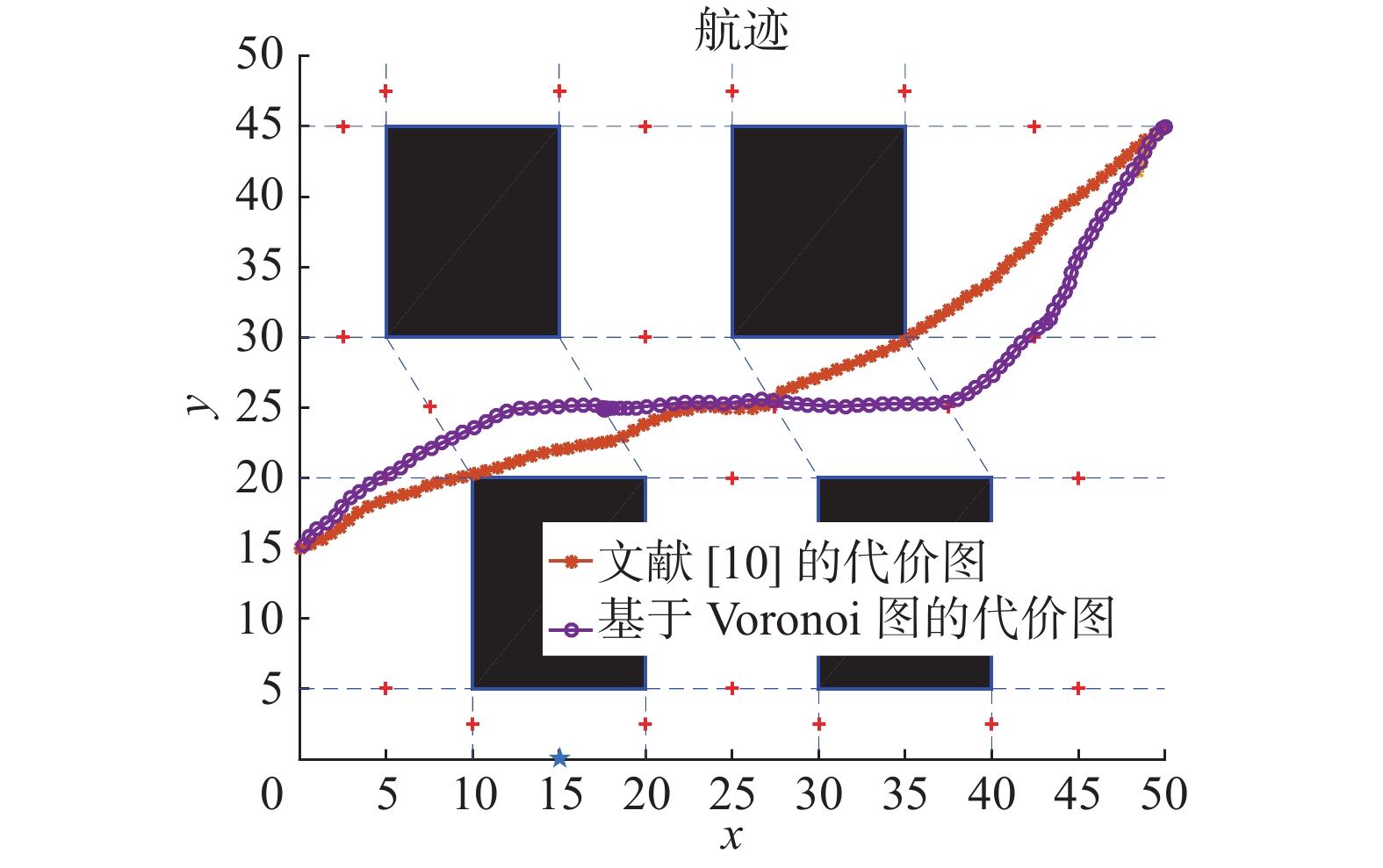Download: 图 4 基于不同代价图航迹规划示意图 Fig. 4 The diagram of trajectory planning based on different cost maps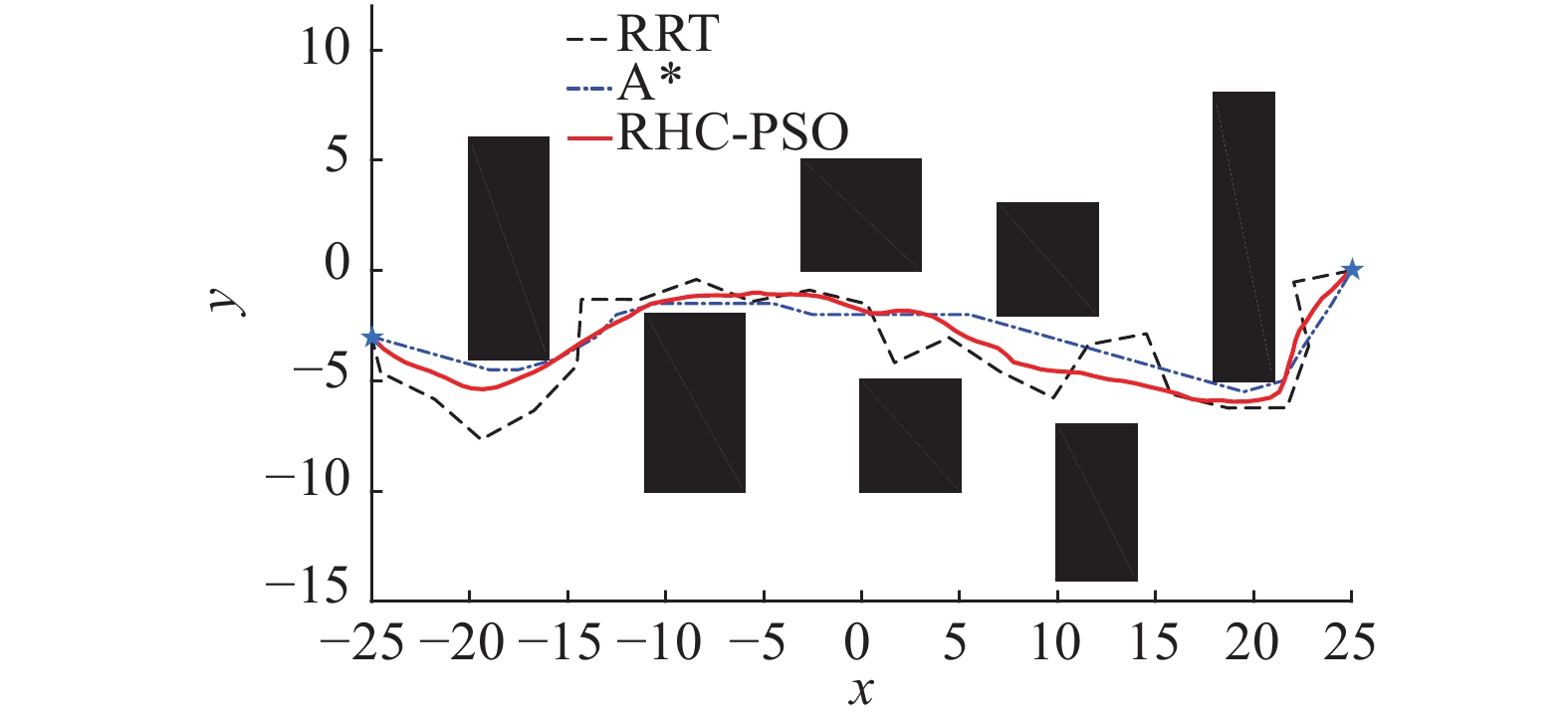Download: 图 5 3种航迹规划方法对比图 Fig. 5 Comparison of three trajectory planning methods表 2 航迹规划比较 Tab.2 Comparison of trajectory planning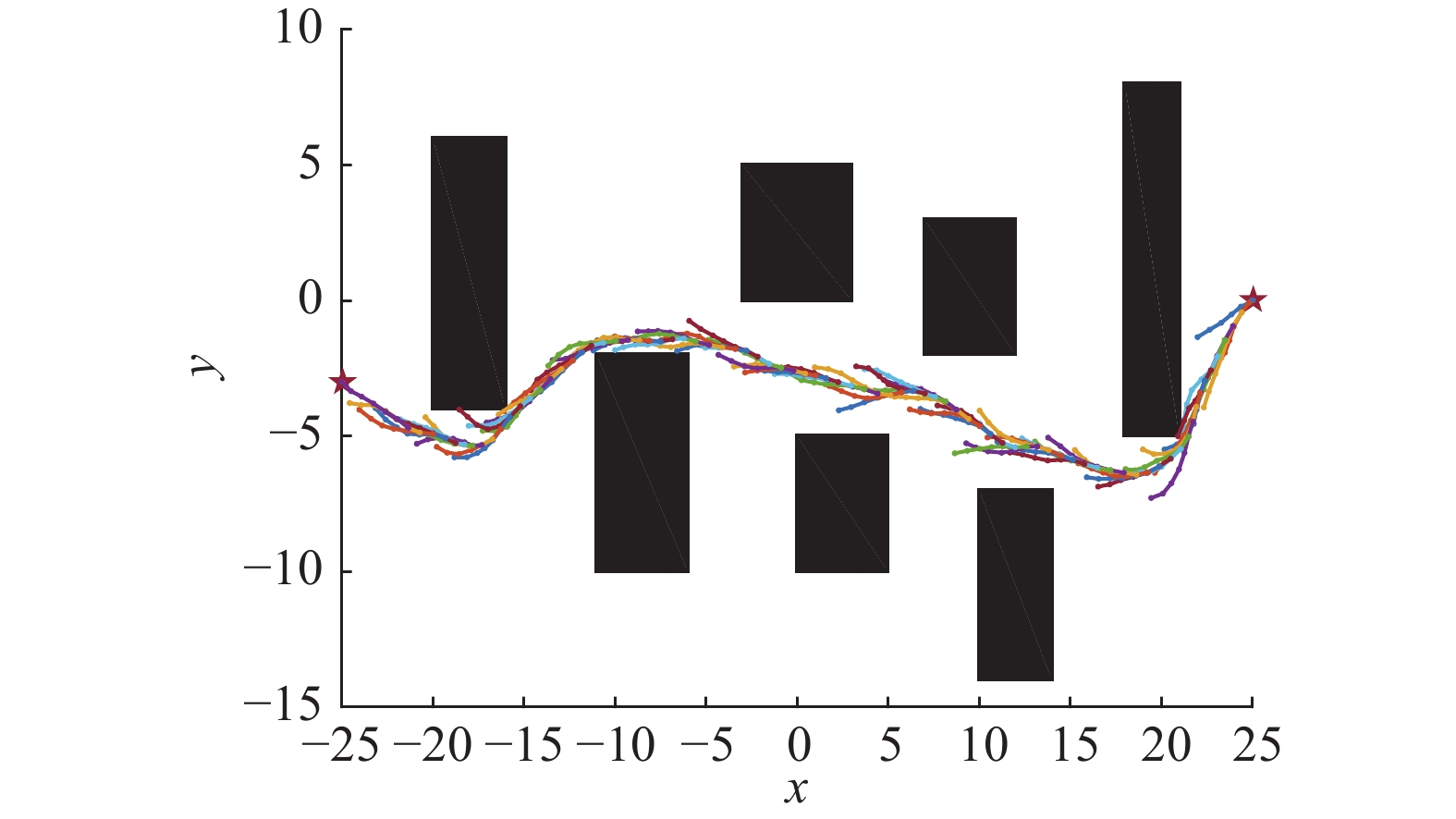Download: 图 6 RHC-FPSO的预测航迹示意图 Fig. 6 The prediction of trajectory based on RHC-FPSO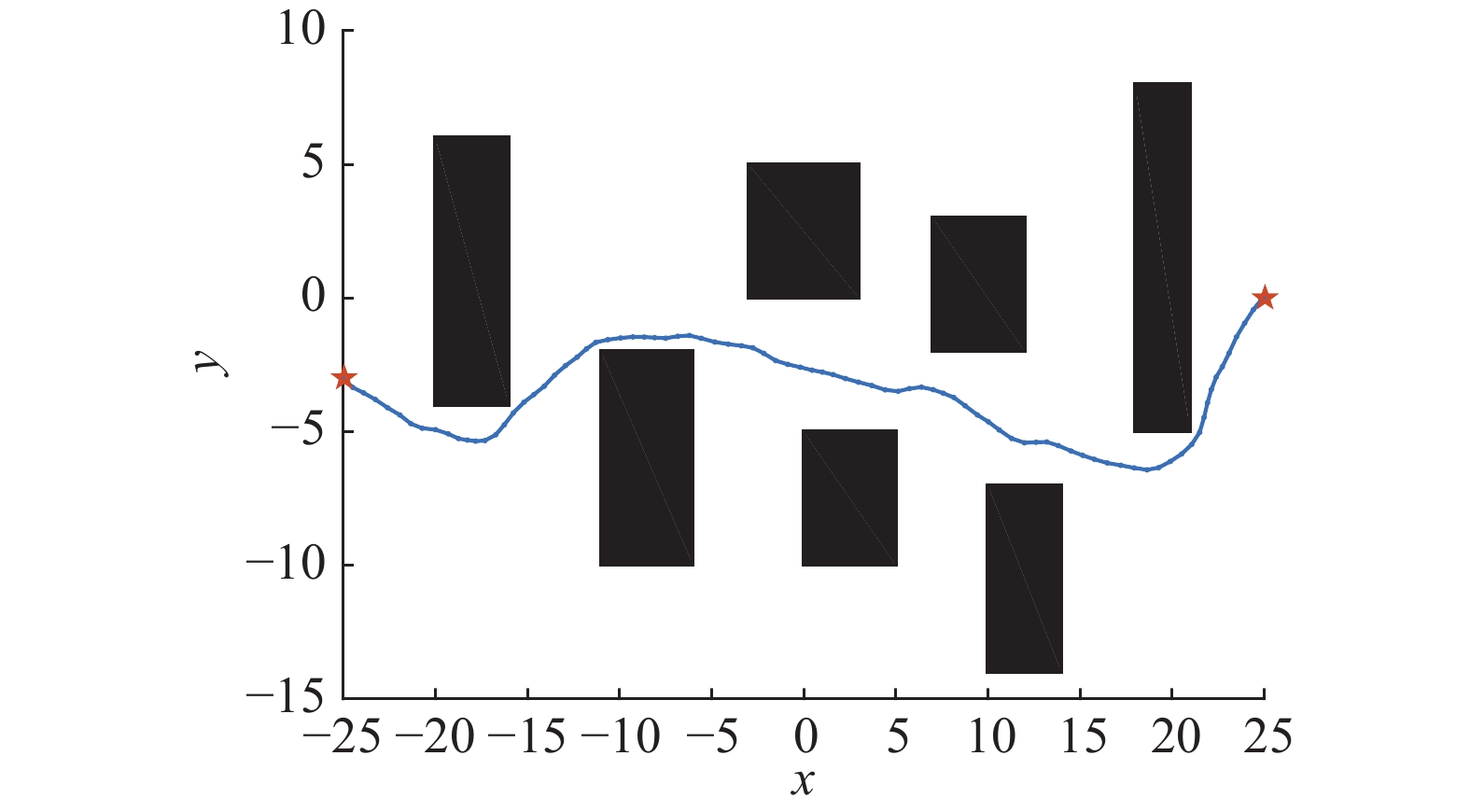Download: 图 7 RHC-FPSO的航迹 Fig. 7 The trajectory based on RHC-FPSO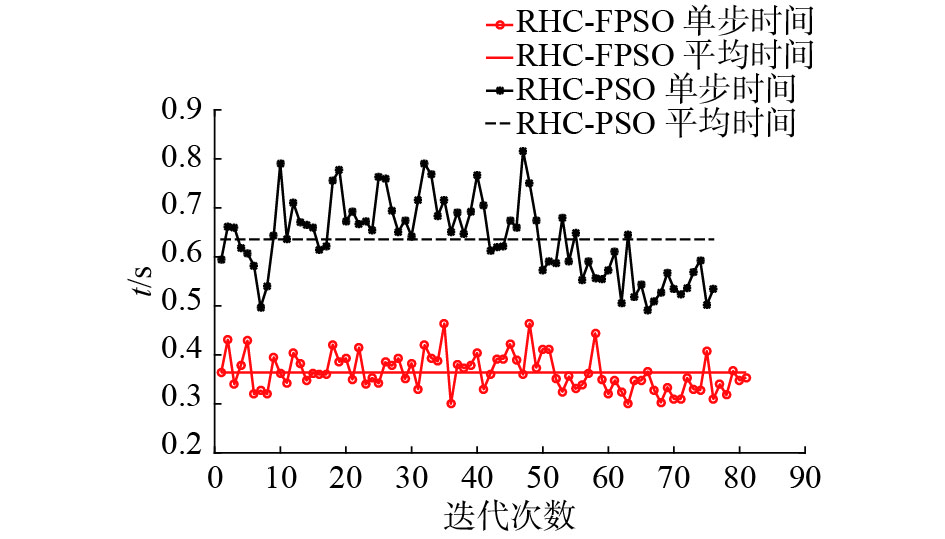Download: 图 8 时间对比图 Fig. 8 Comparison of time

 ${\rm{Stab}} = (\sum\limits_{k = 1}^{N - 1} {\left\| {{{u}}(*|k) - {{u}}(*|k + 1)} \right\|} )/(N - 1)$ (22)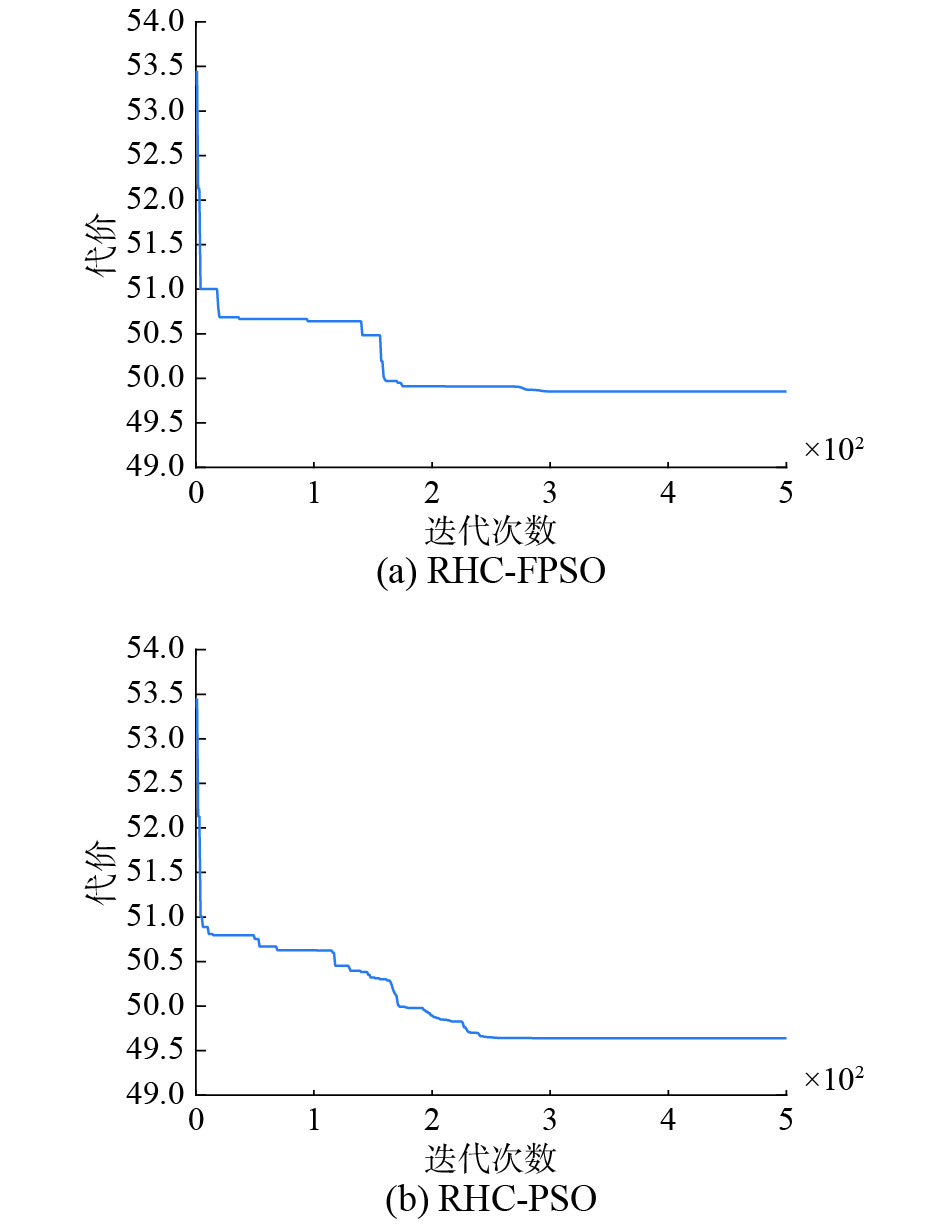Download: 图 9 代价迭代收敛对比 Fig. 9 The convergence comparison of the costs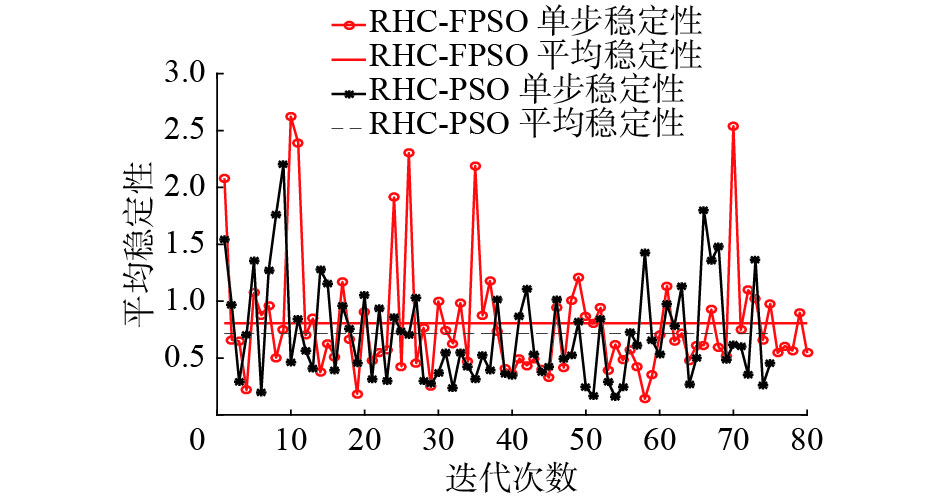Download: 图 10 稳定性对比图 Fig. 10 Comparison of stability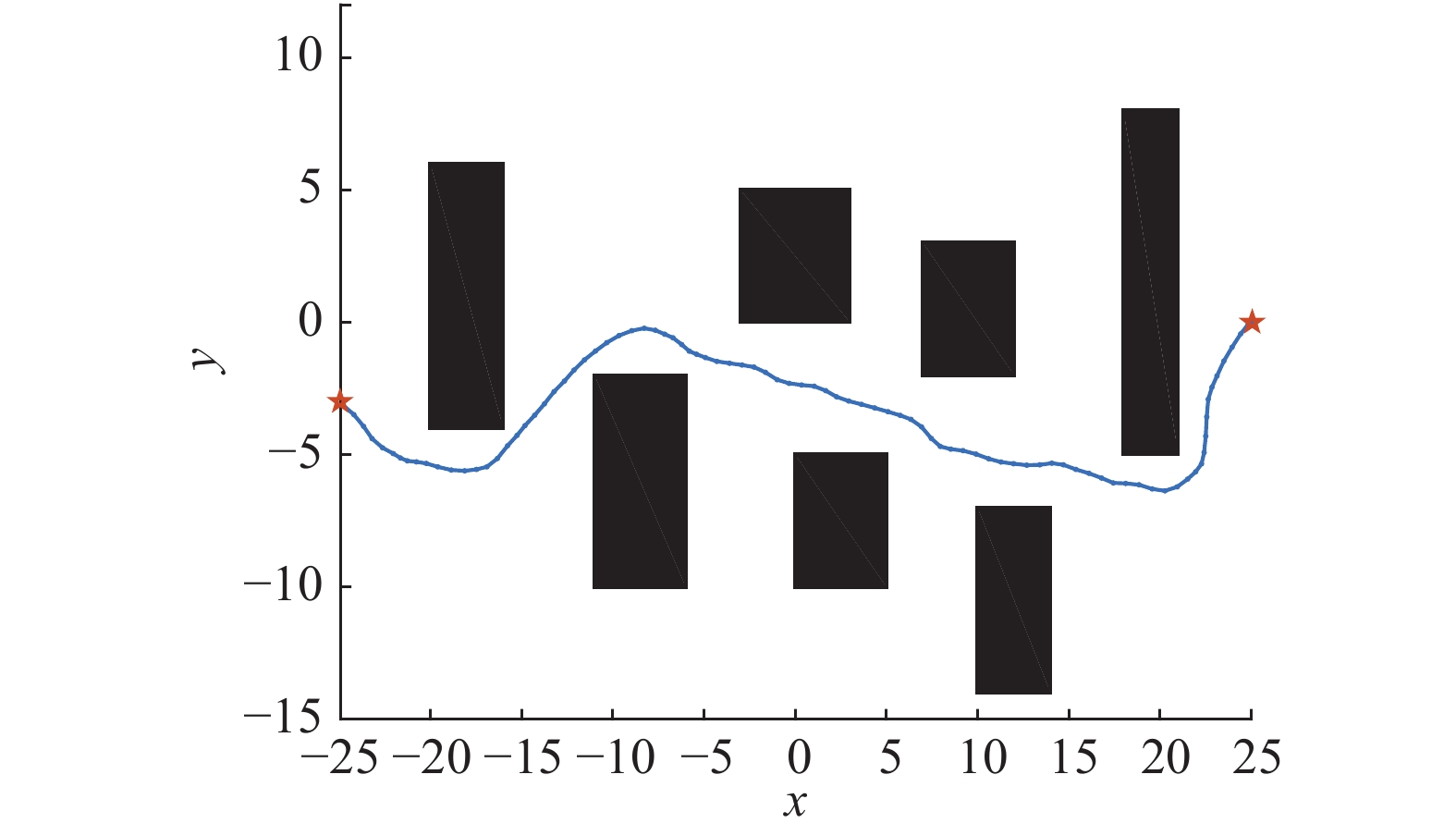Download: 图 11 RHC-APF的航迹 Fig. 11 The trajectory based on RHC-APF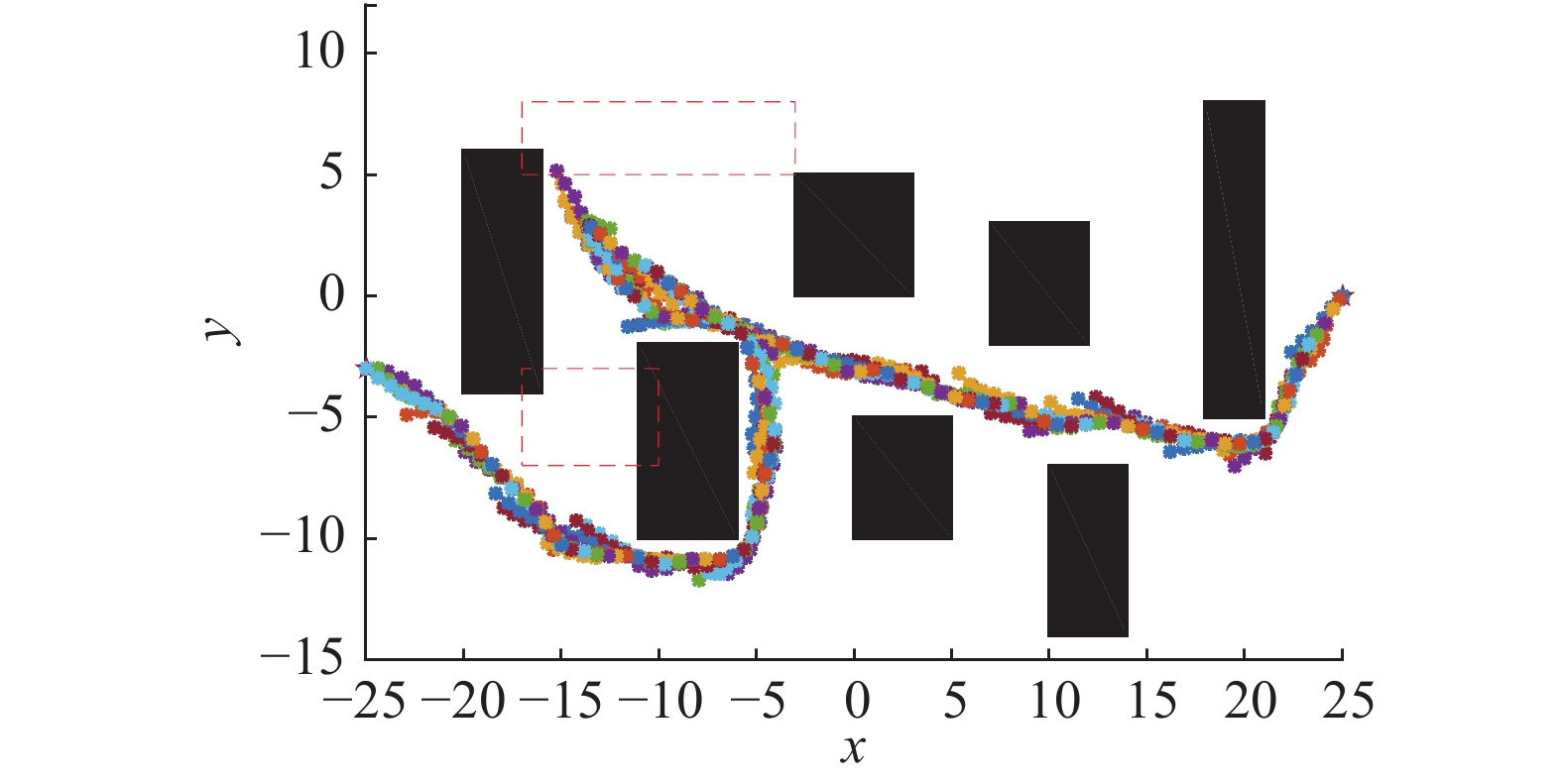Download: 图 12 动态航迹规划预测航迹示意图 Fig. 12 The prediction of dynamic trajectory planning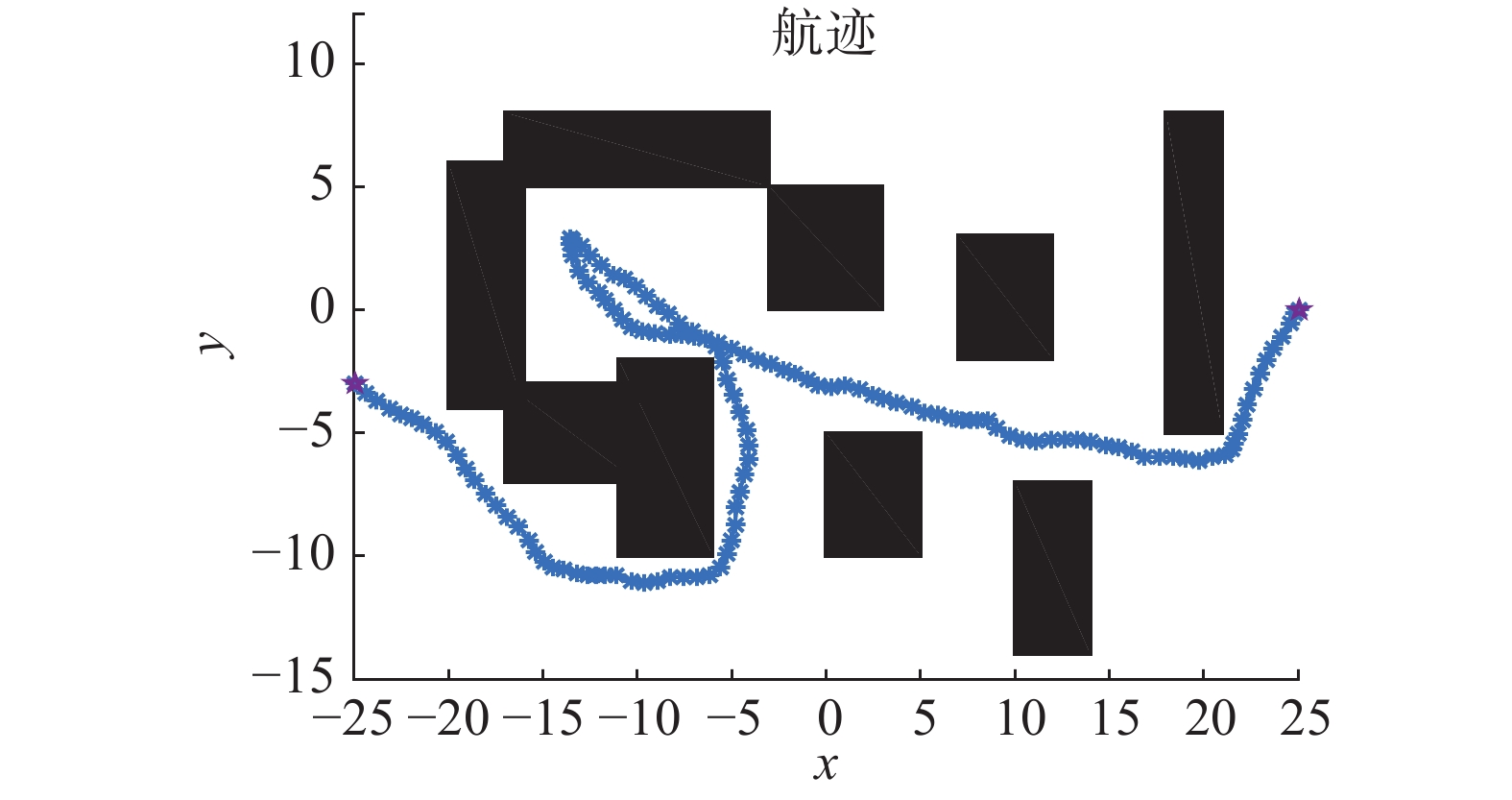Download: 图 13 动态航迹规划 Fig. 13 The dynamic trajectory planning
5 结束语

  郑昌文, 严平, 丁明跃, 等. 飞行器航迹规划[M]. 北京: 国防工业出版社, 2008. ZHENG Changwen, YAN Ping, DING Mingyue, et al. Route planning for air vehicles[M]. Beijing: National Defense Industry Press, 2008. (0)  LEE M C, PARK M G. Artificial potential field based path planning for mobile robots using a virtual obstacle concept[C]//Proceedings of the 2003 IEEE/ASME International Conference on Advanced Intelligent Mechatronics, 2003. Kobe, Japan, 2003: 735–740. (0)  GARCIA M A P, MONTIEL O, CASTILLO O, et al. Path planning for autonomous mobile robot navigation with ant colony optimization and fuzzy cost function evaluation[J]. Applied soft computing, 2009, 9(3): 1102-1110. DOI:10.1016/j.asoc.2009.02.014 (0)  KAVRAKI L E, SVESTKA P, LATOMBE J C, et al. Probabilistic roadmaps for path planning in high-dimensional configuration spaces[J]. IEEE transactions on robotics and automation, 1996, 12(4): 566-580. DOI:10.1109/70.508439 (0)  PHARPATARA P, HÉRISSÉ B, BESTAOUI Y. 3-D trajectory planning of aerial vehicles using RRT[J]. IEEE transactions on control systems technology, 2017, 25(3): 1116-1123. DOI:10.1109/TCST.2016.2582144 (0)  XIAO Hanzhen, LI Zhijun, YANG Chenguang, et al. Robust stabilization of a wheeled mobile robot using model predictive control based on neurodynamics optimization[J]. IEEE transactions on industrial electronics, 2017, 64(1): 505-516. DOI:10.1109/TIE.2016.2606358 (0)  LIU Yang, YU Shuyou, GUO Yang, et al. Receding horizon control for path following problems of wheeled mobile robots[J]. Control theory and application, 2017, 34(4): 424-432. (0)  BELLINGHAM J, RICHARDS A, HOW J P. Receding horizon control of autonomous aerial vehicles[C]//Proceedings of the 2002 American Control Conference. Anchorage, AK, USA, 2002: 3741–3746. (0)  KUWATA Y, SCHOUWENAARS T, RICHARDS A, et al. Robust constrained receding horizon control for trajectory planning[C]//AIAA Guidance, Navigation, and Control Conference and Exhibit, Guidance, Navigation, and Control and Co-located Conferences. San Francisco, 2005: 6079–6091. (0)  RICHARDS A, HOW J P. Aircraft trajectory planning with collision avoidance using mixed integer linear programming[C]//Proceedings of the 2002 American Control Conference. Anchorage, AK, USA, 2002: 1936–1941. (0)  张航, 王琦. 基于RHC的无人机自主轨迹优化算法研究[J]. 航空计算技术, 2007, 37(4): 67-70. ZHANG Hang, WANG Qi. Study on trajectory optimization algorithm for UAV using receding horizon control[J]. Aeronautical computing technique, 2007, 37(4): 67-70. (0)  张胜祥. 基于滚动时域MILP的小型无人机航迹规划[D]. 广州: 华南理工大学, 2009. ZHANG Shengxiang. Path planning of small-scale unmanned helicopters using receding horizon MILP[D]. Guangzhou, China: South China University of Technology, 2009. (0)  李大东, 孙秀霞, 孙彪, 等. 基于混合整数线性规划的无人机任务规划[J]. 飞行力学, 2010, 28(5): 88-91. LI Dadong, SUN Xiuxia, SUN Biao, et al. Mission planning for UAVs based on MILP[J]. Flight dynamics, 2010, 28(5): 88-91. (0)  KUWATA Y, RICHARDS A, SCHOUWENAARS T, et al. Decentralized robust receding horizon control for multi-vehicle guidance[C]//2006 American Control Conference. Minneapolis, MN, USA, 2006: 2047–2052. (0)  ZHAO Jiang, ZHOU Rui. Distributed three-dimensional cooperative guidance via receding horizon control[J]. Chinese journal of aeronautics, 2016, 29(4): 972-983. DOI:10.1016/j.cja.2016.06.011 (0)  ZHANG Yu, WANG Chao, GU Xueqiang, et al. Cooperative trajectory planning for multiple UAVs using distributed receding horizon control and inverse dynamics optimization method[C]//Information Technology and Intelligent Transportation Systems: Volume 1, Proceedings of the 2015 International Conference on Information Technology and Intelligent Transportation Systems ITITS 2015. Xi’an China, 2017: 39–53. (0)  张涛, 于雷, 周中良, 等. 基于混合算法的空战机动决策[J]. 系统工程与电子技术, 2013, 35(7): 1445-1450. ZHANG Tao, YU Lei, ZHOU Zhongliang, et al. Decision-making for air combat maneuvering based on hybrid algorithm[J]. Systems engineering and electronics, 2013, 35(7): 1445-1450. (0)  PENG Z, LI Bo, CHEN Xiaotian, et al. Online route planning for UAV based on model predictive control and particle swarm optimization algorithm[C]//2012 10th World Congress on Intelligent Control and Automation (WCICA). Beijing, China, 2012: 397–401. (0)  钱积新, 赵均, 徐祖华. 预测控制[M]. 北京: 化学工业出版社, 2007. QIAN Jixin, ZHAO Jun, XU Zuhua. Predictive control[M]. Beijing: Chemical Industry Press, 2007. (0)  KHATIB O. Real-time obstacle avoidance for manipulators and mobile robots[J]. The international journal of robotics research, 1986, 5(1): 90-98. DOI:10.1177/027836498600500106 (0)  CORMEN T H, LEISERSON C E, RIVEST R L, et al. Introduction to algorithms[M]. 3rd ed. Cambridge: MIT Press, 2009. (0)  DE BERG M, CHEONG O, VAN KREVELD M, et al. Computational Geometry: Algorithms and Applications[M]. 3rd ed. Berlin Heidelberg: Springer, 2008. (0)